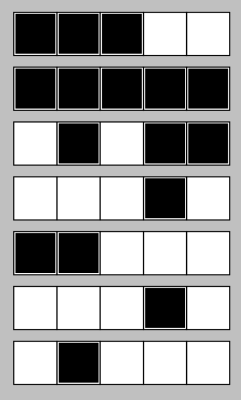### Problem Statement

(Note: This problem statement contains images that should best be viewed in the arena applet.)

You are given N strips of paper. Each strip is a 5 by 1 rectangle, divided into 5 unit squares. Each unit square is either black or white.

Your goal is to arrange all of these strips into a bitmap of height 5 and width N in such a way that the largest possible number appears. Strips can be rotated, and each strip can be placed either horizontally or vertically. Obviously, no two strips may overlap.

We will now explain your goal in more detail. First, we will define the bitmap representations of all decimal digits. Each digit is a bitmap of width 3 and height 5. Below, letters X represent black squares and periods represent white squares.

```..X   XXX   XXX   X.X   XXX
..X   ..X   ..X   X.X   X..
..X   XXX   XXX   XXX   XXX
..X   X..   ..X   ..X   ..X
..X   XXX   XXX   ..X   XXX

XXX   XXX   XXX   XXX   XXX
X..   ..X   X.X   X.X   X.X
XXX   ..X   XXX   XXX   X.X
X.X   ..X   X.X   ..X   X.X
XXX   ..X   XXX   XXX   XXX
```

The bitmap representation of a multiple-digit number consists of the bitmap representations of its digits. Additionally, each pair of consecutive digits is separated by one column of white squares. For example, below is the bitmap representation of the number 47.

```X.X.XXX
X.X...X
XXX...X
..X...X
..X...X
```

The value of N will be one of 3, 7, 11, and 15. Your goal is to assemble a 1-digit number if N=3, a 2-digit number if N=7, a 3-digit number if N=11, or a 4-digit number if N=15. If the number you are trying to assemble has more than one digit, it must not have a leading zero.

You will be given a String[] pieces, describing the N strips of paper you have. Find and return the largest number that can be assembled from these pieces. If there is no such number, return -1 instead.

### Definition

 Class: Digsaw Method: largestNumber Parameters: String[] Returns: int Method signature: int largestNumber(String[] pieces) (be sure your method is public)

### Constraints

-pieces will contain exactly N elements, where N is one of 3, 7, 11, and 15.
-Each element in pieces will have exactly 5 characters, and each of these characters will either be an uppercase letter X or a period.

### Examples

0)

 `{"XXX.X","XXX.X","X.X.X"}`
`Returns: 5`
 Using these three strips, we can assemble either the digit 2 or the digit 5. We return the largest possible answer.
1)

 `{"XXX..","XXXX.","X..X."}`
`Returns: -1`
 No number can be assembled from these strips.
2)

 `{"XXXXX","XXXXX","XXXXX","XXXXX","X...X","X...X","....."}`
`Returns: -1`
 "00" is not a valid 2-digit number, and nothing else can be assembled.
3)

 `{"XXX..","XXXXX",".X.XX","...X.","XX...","...X.",".X..."}`
`Returns: 47`Using these seven strips we can assemble the number 47 as shown in the picture below:4)

 `{"XXXXX","X...X","XXXXX"}`
`Returns: 0`
 Zero is a valid answer in case of one-digit numbers.

#### Problem url:

http://www.topcoder.com/stat?c=problem_statement&pm=10057

#### Problem stats url:

http://www.topcoder.com/tc?module=ProblemDetail&rd=13527&pm=10057

misof

#### Testers:

PabloGilberto , Olexiy , ivan_metelsky , zhuzeyuan

#### Problem categories:

Brute Force, Search